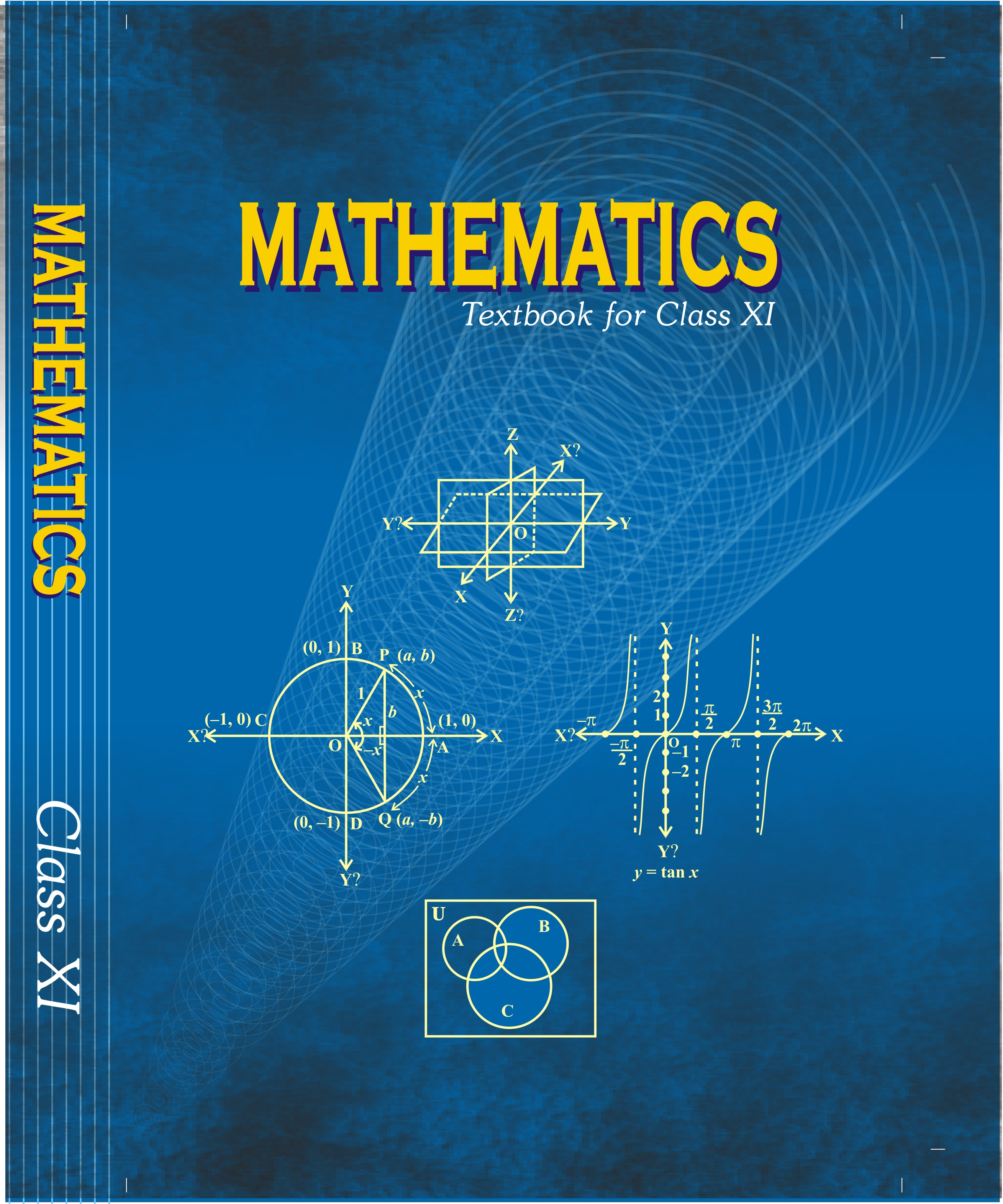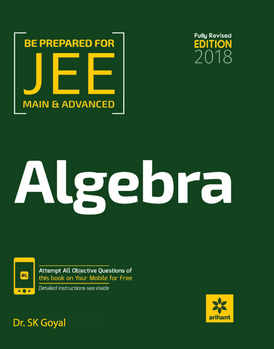# Matrices and Determinants (Weightage 7%)   Share

### Topics from Matrices and Determinants

• Matrices, algebra of matrices, types of matrices (56 concepts)
• Adjoint and evaluation of inverse of a square matrix using deteminants and elementary transformations (14 concepts)
• Test of consistency and solution of simultaneous linear equation in two or three variables using determinants and matrices (15 concepts)
• Determinants and matrices of order two and three (2 concepts)
• Properties of determinants, evaluation of determinants (14 concepts)
• Matrices and Types of Matrices (4 concepts)
• Mathematical Operation on Matrices (5 concepts)
• Special Matrices and their Properties (11 concepts)
• Elementary Row Operations and Inverse of a Matrix by Elementary Row Operations (3 concepts)
• Determinant (2 concepts)
• Adjoint of Matrix (4 concepts)
• Inverse of a Matrix (Using Adjoint) (5 concepts)
• Multiplication of Determinant (1 concepts)
• Properties of Determinants (3 concepts)
• System of Simultaneous Linear equations (4 concepts)

### Important Books for Matrices and Determinants

••Exams
Articles
Questions